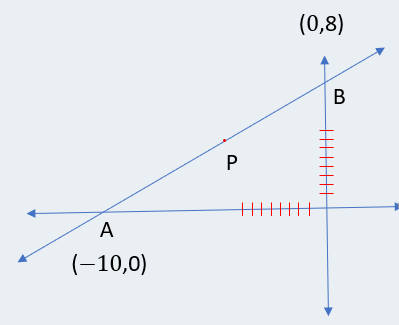# IPMAT Question Paper 2020 | IPM Indore Quants

###### IPMAT Sample Paper | IPMAT Question Paper | Question 13

IPMAT 2020 Question Paper IPM Indore Quantitative Ability. Solve questions from IPMAT 2020 Question Paper from IPM Indore and check the solutions to get adequate practice. The best way to ace IPMAT is by solving IPMAT Question Paper. To solve other IPMAT Sample papers, go here: IPM Sample Paper

Question 13 : The equation of the straight line passing through the point M (-5,1), such that the portion of it between the axes is divided by the point M in to two equal halves, is

1. 10y - 8x = 80
2. 8y + 10x = 80
3. 10y + 8x = 80
4. 8y + 10x + 80 = 0

## Best CAT Coaching in Chennai

#### CAT Coaching in Chennai - CAT 2022Limited Seats Available - Register Now!

The point P divides AB into 2 halves.
AP = PB
A is on the x axis, Hence Point A (h,0)
B is on the Y axis, Hence Point B (0,k)We need to use midpoint formula.
[$$frac{$h + 0$}{2}) , $$frac{$k + 0$}{2})] = (-5 , 4)
$$frac{h}{2}$ = - 5 h = -10 $\frac{k}{2} = 4 k = 8Hence the Y intercept is 8 and X intercept is - 10. \\frac{x}{-10}$ + $\frac{y}{8}$ = 1 -8x + 10y = 80 The equation is -8x + 10y = 80 The question is " The equation of the straight line passing through the point M$-5,1), such that the portion of it between the axes is divided by the point M in to two equal halves, is "

##### Hence, the answer is 10y - 8x = 80

Choice A is the correct answer.

##### Where is 2IIM located?

2IIM Online CAT Coaching
A Fermat Education Initiative,
58/16, Indira Gandhi Street,
Kaveri Rangan Nagar, Saligramam, Chennai 600 093

##### How to reach 2IIM?

Mobile: (91) 99626 48484 / 94459 38484
WhatsApp: WhatsApp Now
Email: info@2iim.com